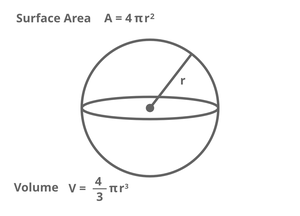# Java Program to Find the Volume and Surface Area of Sphere

• Last Updated : 26 Nov, 2020

A sphere is a geometrical object in 3D space that is the surface of a ball. The sphere is defined mathematically as the set of points that are all at the same distance of radius from a given point in a 3D space.Example:

```Input:     radius = 5
Output:    Surface Area ≈ 314.16
Volume ≈ 523.6

Output:    Surface Area ≈ 113.1
Volume ≈ 113.1```

Surface area of  a sphere = 4*3.14*(r*r)

Volume of a sphere = (4/3)*3.14*(r*r*r)

Algorithm

1. Initializing value of r as 5.0, surface area as 0.0,volume as 0.0
2. Calculating surface area and volume of a sphere using the below formulas
3. Surface area=4*3.14(r*r)
4. Volume=(4/3)3.14(r*r*r)
5. Display surface area and volume

Implementation:

## Java

 `// Java Program to Find the Volume and Surface Area of``// Sphere``class` `surfaceareaandvolume {``    ``public` `static` `void` `main(String[] args)``    ``{``        ``double` `r = ``5.0``, surfacearea = ``0.0``, volume = ``0.0``;``        ``surfacearea = ``4` `* ``3.14` `* (r * r);``        ``volume = ((``double``)``4` `/ ``3``) * ``3.14` `* (r * r * r);``       ` `        ``System.out.println(``"surfacearea of sphere ="``                           ``+ surfacearea);``       ` `        ``System.out.println(``"volume of sphere ="` `+ volume);``    ``}``}`

Output

```surfacearea of sphere =314.0
volume of sphere =523.3333333333334```

Time Complexity: O(1)

Space Complexity: O(1)

My Personal Notes arrow_drop_up